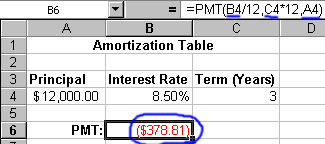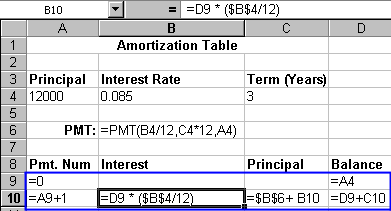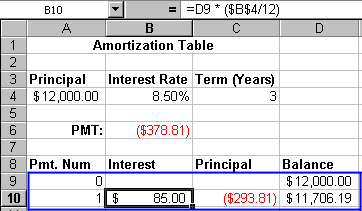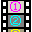### Lesson 10: A Useful Example – A Loan Amortization Table

#### How do you create an amortization table using the Pmt function?

This lesson guides you through the process of creating an amortization table with Microsoft Excel. It's a useful example because an amortization table can help you make wise decisions about borrowing money or repaying loans. An amortization table shows for each payment of a loan how much of the payment is going toward the interest on the unpaid balance and how much is going toward the principal.

This lesson explains how to create an amortization table. Before continuing you should read the related concept which explains what an amortization table is.

Creating an amortization table is a 3 step process:

1. Use the =PMT function to calculate the monthly payment.
2. Create the first two lines of your table using formulas with the correct relative and absolute references.
3. Use the Fill Down feature of Excel to create the rest of the table.

Step 1
The key to creating an amortization table is the PMT function. The PMT function is a built-in function that computes the periodic payment given the principal, rate, and term. Here is the syntax:

=PMT(rate, term, principal)

For example, if you borrow \$12,000 at 8.5% for 3 years, your monthly payment would be:

=PMT(.085/12,3*12,12000) = \$-378.81

Notes:

• The result is negative because it represents money you are paying.
• The values for rate and term must be consistent. If you are making a payment each month, the term is the number of months and the rate is the interest rate for one month.

Using the PMT function and a few simple formulas, you can generate an amortization table.

The first step is to specify the terms of the loan and use the PMT function to calculate the monthly payment.Figure 1. Using the =PMT() function

Notice that in the above example the parameters to the PMT function are cell references. This allows you to change a value in row 4 and quickly see how your change effects the monthly payment--especially handy when you are doing what-if analysis. Also notice that the value in B6 is displayed in red and in parenthesis. This is the default format for displaying negative numbers.

Step 2
In this step you add the first two lines of the table. Using the View Formulas feature of Excel, the following figure shows the formulas in the first two lines of our amortization table:Figure 2. First two lines of an amortization table with formulas showing

Figure 3 below shows the values you would see given the above formulas.Figure 3. First two lines with values showing

Pmt. Num (Payment Number)
starts at 0 and is incremented for each payment.
Interest
is the loan balance multiplied by the interest rate for the compounding period. The interest rate in cell B6 is the interest rate per year. We divide by 12 to get the interest owed for one month. Note the absolute reference \$B\$4. This keeps the reference from changing during the fill down step that's coming up shortly.
Principal
can be calculated by adding the interest paid for the current period to the value in cell B6 (the monthly payment). It might seem odd that we are adding. Take a close look at the value in cell B6. It's negative. When you add a positive number (B10) to a negative number (B6) you get a less negative number.
Balance
is the previous balance minus the principal paid. Since the principal paid is a negative number we add it to the balance.

Step 3
The relative references in row 10 refer to other terms in the amortization table so we can use the Fill Down command to fill out the other rows in the table. The avi movie below demonstrates how to use the Fill Down command. In short,

1. Click and drag the mouse cursor across row 10 to highlight cells A10 - D10.
2. Now click and drag down the handle at the lower right of the border surrounding the highlighted area.
3. When you release the mouse the row you highlighted will be copied down to fill out your table.If you would like a copy of the spreadsheet developed here it is available for download from this page. Both the Microsoft Excel and Microsoft Works versions are available.

 Copyright 1999 by the Curators of the University of Missouri